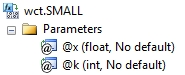# SQL Server function to calculate kth smallest value in a dataset

SMALL

Updated: 28 February 2011

Note: This documentation is for the SQL2008 (and later) version of this XLeratorDB function, it is not compatible with SQL Server 2005.

Use the aggregate function SMALL to calculate the kth smallest value in a dataset. Use this function to return a value based on its relative standing.
SyntaxArguments
@x
the input values for the function. @ x is an expression of type float or of a type that can be implicitly converted to float.
@k
is the position (from the smallest) in the dataset to return. @k is an expression of type float or of a type that can be implicitly converted to float.
Return Types
float
Remarks
·         If @k = 0 or @k is greater than the number of rows in the dataset, SMALL returns an error.
·         If n is the number of rows in the dataset then @k = 1 returns the smallest value in the dataset and @k = n returns the largest value in the dataset.
·         @k must remain invariant for a group.
·         SMALL is an aggregate function and follows the same conventions as all other aggregate functions in SQL Server.
·         If you have previously used the SMALL scalar function, the SMALL aggregate has a different syntax. The SMALL scalar function is no longer available in XLeratorDB/statistics2008, though you can still use the scalar SMALL _q
Examples
In this example we will select the smallest value.
SELECT wct.SMALL(
x     --@x
,1    --@k
) as SMALL
FROM (VALUES
(1),(2),(2),(2),(2),(3),(4),(5),(6),(7)
,(8),(8),(8),(8),(9),(10),(11),(12),(13)
,(13),(14)
) n(x)

This produces the following result
SMALL
----------------------
1

(1 row(s) affected)

To select the third smallest value in the table,
SELECT wct.SMALL(
x
,3
) as SMALL
FROM (VALUES
(1),(2),(2),(2),(2),(3),(4),(5),(6),(7)
,(8),(8),(8),(8),(9),(10),(11),(12),(13)
,(13),(14)
) n(x)

This produces the following result
SMALL
----------------------
2

(1 row(s) affected)

Since SMALL is an aggregate function, we can take advanatge of the grouping capabilities of aggregates. In this example, we calulate the 5th smallest value in each group.
SELECT grp, wct.SMALL(x, 5) as SMALL
FROM (VALUES
('Group 1',330854662),
('Group 1',-787948950),
('Group 1',104760636),
('Group 1',-99072558),
('Group 1',326055082),
('Group 1',401353001),
('Group 1',143405916),
('Group 1',420320581),
('Group 1',-743843531),
('Group 1',839451820),
('Group 2',-196956602),
('Group 2',-578645024),
('Group 2',31690798),
('Group 2',332317230),
('Group 2',973774976),
('Group 2',-263698684),
('Group 2',645094628),
('Group 2',718352395),
('Group 2',-358941981),
('Group 2',447414655),
('Group 3',-435711121),
('Group 3',-490640272),
('Group 3',-665860253),
('Group 3',480661704),
('Group 3',-456298282),
('Group 3',437436241),
('Group 3',-6535783),
('Group 3',311292734),
('Group 3',-150346309),
('Group 3',194606550),
('Group 4',995355206),
('Group 4',-794694938),
('Group 4',-310339872),
('Group 4',461478744),
('Group 4',647091760),
('Group 4',-625559637),
('Group 4',-65067110),
('Group 4',477468966),
('Group 4',-765348260),
('Group 4',596374768),
('Group 5',302764911),
('Group 5',226227458),
('Group 5',763226571),
('Group 5',-151647754),
('Group 5',422758913),
('Group 5',-264094908),
('Group 5',914420900),
('Group 5',48940564),
('Group 5',-759334474),
('Group 5',408007858)
)n(grp, x)
GROUP BY grp

This produces the following result.
grp                      SMALL
------- ----------------------
Group 1              143405916
Group 2               31690798
Group 3             -150346309
Group 4              -65067110
Group 5              226227458

(5 row(s) affected)

And, we could even do something like getting the second smallest of the fifth smallest from the groups.
SELECT wct.SMALL(g.y, 2) as SMALL
FROM (
SELECT grp, wct.SMALL(x, 5) as SMALL
FROM (VALUES
('Group 1',330854662),
('Group 1',-787948950),
('Group 1',104760636),
('Group 1',-99072558),
('Group 1',326055082),
('Group 1',401353001),
('Group 1',143405916),
('Group 1',420320581),
('Group 1',-743843531),
('Group 1',839451820),
('Group 2',-196956602),
('Group 2',-578645024),
('Group 2',31690798),
('Group 2',332317230),
('Group 2',973774976),
('Group 2',-263698684),
('Group 2',645094628),
('Group 2',718352395),
('Group 2',-358941981),
('Group 2',447414655),
('Group 3',-435711121),
('Group 3',-490640272),
('Group 3',-665860253),
('Group 3',480661704),
('Group 3',-456298282),
('Group 3',437436241),
('Group 3',-6535783),
('Group 3',311292734),
('Group 3',-150346309),
('Group 3',194606550),
('Group 4',995355206),
('Group 4',-794694938),
('Group 4',-310339872),
('Group 4',461478744),
('Group 4',647091760),
('Group 4',-625559637),
('Group 4',-65067110),
('Group 4',477468966),
('Group 4',-765348260),
('Group 4',596374768),
('Group 5',302764911),
('Group 5',226227458),
('Group 5',763226571),
('Group 5',-151647754),
('Group 5',422758913),
('Group 5',-264094908),
('Group 5',914420900),
('Group 5',48940564),
('Group 5',-759334474),
('Group 5',408007858)
)n(grp, x)
GROUP BY grp
) g(x, y)

This produces the following result.
SMALL
----------------------
-65067110

(1 row(s) affected)

### SupportCopyright 2008-2023 Westclintech LLC         Privacy Policy        Terms of Service